In this article, I am going to discuss Octal to Hexadecimal Conversion with Examples. Please read our previous article where we discussed Octal and Hexadecimal to Binary Conversion as well as Binary to Octal and Hexadecimal Conversion with Examples.

Now let us see the conversion of octal numbers to hexadecimal numbers. There is no direct method for this conversion. One method is to convert octal to decimal and then convert decimal to the hexadecimal number system. Or else, we can also take the help of a binary number system. So, taking the help of binary will be easy for us. So let us see the conversion.

Let us take a number, (276)8, this is an octal number. To convert this into hexadecimal, first, we have to convert this into a binary number system. We have to write all the digits separately,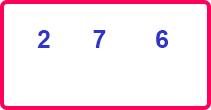Now we will write the corresponding binary figures to all these digits.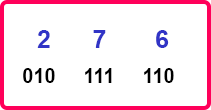So now let us combine these binary figures and form our binary number.

10111110 is the binary number.

(276)8 = (10111110)2

We have converted the octal number to a binary number but we required a hexadecimal number. So now covert the binary number into a hexadecimal number. For this conversion, we have to make bundles of 4 digits from the right-hand side in the above binary number.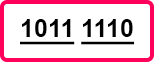Now simply write down the hexadecimal digits for the bundled binary figure. You can write it the help of the table which we have shared in our previous articles.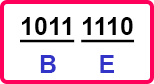(10111110)2 = (BE)16, or (276)8 = (BE)16

So first we have converted octal to binary then binary to hexadecimal. Now let us take one hexadecimal number and convert that into the octal number system.

So, the number is (1C8)16. This is in hexadecimal form. So first, we have to convert it into a binary system. Let us write down the digits,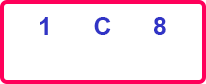Now write the binary figures of all these digits,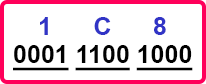111001000 is the binary form. Now we have to convert this binary number into an octal number. So write this binary number in the bundle of 3 digits from the right-hand side.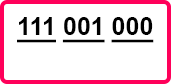Now, write the decimal digits corresponding to the above-bundled pairs of binary digits.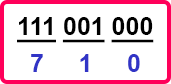So, (1C8)16 = (111001000)2,

(111001000)2 = (710)8

So, these are the methods of conversion from hexadecimal to octal number system and vice-versa. You can practice this by taking some numbers. So that’s all about the number system.